# Non Verbal Reasoning - Analogy

### Exercise :: Analogy - Section 2

Each of the following questions consists of two sets of figures. Figures A, B, C and D constitute the Problem Set while figures 1, 2, 3, 4 and 5 constitute the Answer Set. There is a definite relationship between figures A and B. Establish a similar relationship between figures C and D by selecting a suitable figure from the Answer Set that would replace the question mark (?) in fig. (D).

41.

Select a suitable figure from the Answer Figures that would replace the question mark (?).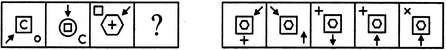(A)     (B)      (C)     (D)                  (1)      (2)      (3)      (4)      (5)

 A. 1 B. 2 C. 3 D. 4 E. 5

Explanation:

The arrow rotates 135oCW and moves a distance equal to one and a half sides of the square boundary in a CW direction. Out of the two central elements, the outer element diminishes in size and becomes the inner element, the inner element moves to the position of the corner element and the corner element gets enlarged and moves to the central position to become the outer element.

42.

Select a suitable figure from the Answer Figures that would replace the question mark (?).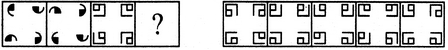(A)     (B)      (C)     (D)                  (1)      (2)      (3)      (4)      (5)

 A. 1 B. 2 C. 3 D. 4 E. 5

Explanation:

The upper-left and the lower-right elements rotate 90oCW while the upper-right and the lower-left elements rotate 90oACW.

43.

Select a suitable figure from the Answer Figures that would replace the question mark (?).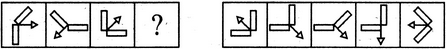(A)     (B)      (C)     (D)                  (1)      (2)      (3)      (4)      (5)

 A. 1 B. 2 C. 3 D. 4 E. 5

Explanation:

The arrow rotates 135oCW and the remaining part of the figure rotates 90oACW.

44.

Select a suitable figure from the Answer Figures that would replace the question mark (?).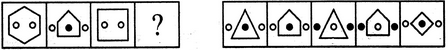(A)     (B)      (C)     (D)                  (1)      (2)      (3)      (4)      (5)

 A. 1 B. 2 C. 3 D. 4 E. 5

Explanation:

The number of sides in the main figure reduces by one; the two circles present inside it, move outside and reach the positions on either side of this figure; a new black circle appears inside the figure.

45.

Select a suitable figure from the Answer Figures that would replace the question mark (?).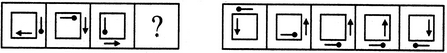(A)     (B)      (C)     (D)                  (1)      (2)      (3)      (4)      (5)

 A. 1 B. 2 C. 3 D. 4 E. 5